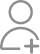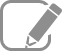Take Class 11 Tuition from the Best Tutors

•Affordable fees
•1-1 or Group class
•Flexible Timings
•Verified Tutors

Search in

# On a two-lane road, car A is travelling with a speed of 36 km h–1. Two cars B and C approach car A in opposite directions with a speed of 54 km h–1 each. At a certain instant, when the distance AB is equal to AC, both being 1 km, B decides to overtake A before C does. What minimum acceleration of car B is required to avoid an accident ?Follow 1AnswerVelocity of car A, vA = 36 km/h = 10 m/s Velocity of car B, vB = 54 km/h = 15 m/s Velocity of car C, vC = 54 km/h = 15 m/s Relative velocity of car B with respect to car A, vBA = vB – vA = 15 – 10 = 5 m/s Relative velocity of car C with respect to car A, vCA = vC – (– vA) = 15...

Velocity of car A, vA = 36 km/h = 10 m/s

Velocity of car B, vB = 54 km/h = 15 m/s

Velocity of car C, vC = 54 km/h = 15 m/s

Relative velocity of car B with respect to car A,

vBA = vB – vA

= 15 – 10 = 5 m/s

Relative velocity of car C with respect to car A,

vCA = v– (– vA)

= 15 + 10 = 25 m/s

At a certain instance, both cars B and C are at the same distance from car A i.e.,

s = 1 km = 1000 m

Time taken (t) by car C to cover 1000 m =Hence, to avoid an accident, car B must cover the same distance in a maximum of 40 s.

From second equation of motion, minimum acceleration (a) produced by car B can be obtained as:Now ask question in any of the 1000+ Categories, and get Answers from Tutors and Trainers on UrbanPro.com

Find Class 11 Tuition near you

Looking for Class 11 Tuition ?

Learn from the Best Tutors on UrbanPro

Are you a Tutor or Training Institute?

Join UrbanPro Today to find students near you
X

### Looking for Class 11 Tuition Classes?

The best tutors for Class 11 Tuition Classes are on UrbanPro

• Select the best Tutor
• Book & Attend a Free Demo
• Pay and start Learning### Take Class 11 Tuition with the Best Tutors

The best Tutors for Class 11 Tuition Classes are on UrbanPro